# PSAT Math : Integers

## Example Questions

### Example Question #1 : How To Subtract Even Numbers

Ifis an odd integer andis an even integer, which of the following must true of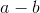?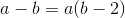The result will be odd.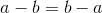The result will be even.

We cannot draw any conclusions from the given information.

The result will be odd.

Explanation:

An even number subtracted from an odd number will always produce an odd result.

None of the other answer choices are correct.

### Example Question #1 : How To Add Even Numbers

If x represents an even integer, which of the following expressions represents an odd integer?

5x + 4

x + 2

2x – 2

3x + 1

3x – 2

3x + 1

Explanation:

Pick any even integer (2, 4, 6, etc.) to represent x. The only value that is odd is 3x + 1. Any number multiplied by an even integer will be even. When an even number is added and subtracted to that product, the result will be even as well. 3x + 1 is the only choice that adds an odd number to the product.

### Example Question #1 : How To Divide Even Numbers

If m and n are both even integers, which of the following must be true?

l. m2/n2 is even

ll. m2/n2 is odd

lll. m2 + n2 is divisible by four

I only

II only

III only

I & III only

none

III only

Explanation:

While I & II can be true, examples can be found that show they are not always true (for example, 22/22 is odd and 42/22 is even).

III is always true – a square even number is always divisible by four, and the distributive property tell us that adding two numbers with a common factor gives a sum that also has that factor.

### Example Question #1 : How To Divide Even Numbers

Let S be a set that consists entirely of even integers, and let T be the set that consists of each of the elements in S increased by two. Which of the following must be even?

I. the mean of T

II. the median of T

III. the range of T

I and II only

I only

II only

II and III only

III only

III only

Explanation:

S consists of all even integers. If we were to increase each of these even numbers by 2, then we would get another set of even numbers, because adding 2 to an even number yields an even number. In other words, T also consists entirely of even numbers.

In order to find the mean of T, we would need to add up all of the elements in T and then divide by however many numbers are in T. If we were to add up all of the elements of T, we would get an even number, because adding even numbers always gives another even number. However, even though the sum of the elements in T must be even, if the number of elements in T was an even number, it's possible that dividing the sum by the number of elements of T would be an odd number.

For example, let's assume T consists of the numbers 2, 4, 6, and 8. If we were to add up all of the elements of T, we would get 20. We would then divide this by the number of elements in T, which in this case is 4. The mean of T would thus be 20/4 = 5, which is an odd number. Therefore, the mean of T doesn't have to be an even number.

Next, let's analyze the median of T. Again, let's pretend that T consists of an even number of integers. In this case, we would need to find the average of the middle two numbers, which means we would add the two numbers, which gives us an even number, and then we would divide by two, which is another even number. The average of two even numbers doesn't have to be an even number, because dividing an even number by an even number can produce an odd number.

For example, let's pretend T consists of the numbers 2, 4, 6, and 8. The median of T would thus be the average of 4 and 6. The average of 4 and 6 is (4+6)/2 = 5, which is an odd number. Therefore, the median of T doesn't have to be an even number.

Finally, let's examine the range of T. The range is the difference between the smallest and the largest numbers in T, which both must be even. If we subtract an even number from another even number, we will always get an even number. Thus, the range of T must be an even number.

Of choices I, II, and III, only III must be true.

### Example Question #1 : How To Multiply Even Numbers

If x is an even integer and y is an odd integer. Which of these expressions represents an odd integer?

I. xy

II. x-y

III. 3x+2y

I and II only

II and III only

II only

I and III only

I, II, and III only

II only

Explanation:

I)xy is Even*Odd is Even. II) x-y is Even+/-Odd is Odd. III) 3x is Odd*Even =Even, 2y is Even*Odd=Even, Even + Even = Even. Therefore only II is Odd.

### Example Question #21 : Even / Odd Numbers

If x is an even number, y is an odd number, and z is an even number, which of the following will always give an even number?

I. xyz

II. 2x+3y

III. z– y

II only

I and II only

I only

I, II and III

II and III only

I only

Explanation:

I. xyz = even * odd * even = even

II. 2x + 3y = even*even + odd*odd = even + odd = odd

III. z– y = even * even – odd = even – odd = odd

Therefore only I will give an even number.

### Example Question #1 : How To Multiply Even Numbers

If x and are integers and at least one of them is even, which of the following MUST be true?

x + y is odd

xy is odd

xis even

xy is even

Nothing can be determined based on the given information

xy is even

Explanation:

Since we are only told that "at least" one of the numbers is even, we could have one even and one odd integer OR we could have two even integers.

Even plus odd is odd, but even plus even is even, so xy could be either even or odd.

Even times odd is even, and even times even is even, so xy must be even.

### Example Question #1551 : Sat Mathematics

Let a and b be positive integers such that ab2 is an even number. Which of the following must be true?

I. ais even

II. a2b is even

III. ab is even

II and III only

I and II only

I only

I, II, and III

II only

II and III only

Explanation:

In order to solve this problem, it will help us to find all of the possible scenarios of a, b, a2, and b2. We need to make use of the following rules:

1. The product of two even numbers is an even number.

2. The product of two odd numbers is an odd number.

3. The product of an even and an odd number is an even number.

The information that we are given is that ab2 is an even number. Let's think of ab2 as the product of two integers: a and b2.

In order for the product of a and b2 to be even, at least one of them must be even, according to the rules that we discussed above. Thus, the following scenarios are possible:

Scenario 1: a is even and b2 is even

Scenario 2: a is even and b2 is odd

Scenario 3: a is odd and b2 is even

Next, let's consider what possible values are possible for b. If b2 is even, then this means b must be even, because the product of two even numbers is even. If b were odd, then we would have the product of two odd numbers, which would mean that b2 would be odd. Thus, if b2 is even, then b must be even, and if b2 is odd, then b must be odd. Let's add this information to the possible scenarios:

Scenario 1: a is even, b2 is even, and b is even

Scenario 2: a is even, b2 is odd, and b is odd

Scenario 3: a is odd, b2 is even, and b is even

Lastly, let's see what is possible for a2. If a is even, then amust be even, and if a is odd, then a2 must also be odd. We can add this information to the three possible scenarios:

Scenario 1: a is even, b2 is even, and b is even, and a2 is even

Scenario 2: a is even, b2 is odd, and b is odd, and a2 is even

Scenario 3: a is odd, b2 is even, and b is even, and a2 is odd

Now, we can use this information to examine choices I, II, and III.

Choice I asks us to determine if a2 must be even. If we look at the third scenario, in which a is odd, we see that a2 would also have to be odd. Thus it is possible for a2 to be odd.

Next, we can analyze a2b. In the first scenario, we see that a2 is even and b is even. This means that a2b would be even. In the second scenario, we see that a2 is even, and b is odd, which would still mean that a2b is even. And in the third scenario, a2 is odd and b is even, which also means that a2b would be even. In short, a2b is even in each of the possible scenarios, so it must always be even. Thus, choice II must be true.

We can now look at ab. In scenario 1, a is even and b is even, which means that ab would also be even. In scenario 2, a is even and b is odd, which means that ab is even again. And in scenario 3, a is odd and b is even, which again means that ab is even. Therefore, ab must be even, and choice III must be true.

The answer is II and III only.

### Example Question #1 : How To Multiply Even Numbers

Let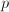equal the product of two numbers.  If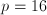, then the two numbers COULD be which of the following?

8 and 8

32 and 2

0 and 16

20 and 4

2 and 8

2 and 8

Explanation:

The word "product" refers to the answer of a multiplication problem.  Since 2 times 8 equals 16, it is a valid pair of numbers.

### Example Question #29 : Integers

If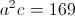andis an odd integer, which of the following couldbe divisible by?Ifis an odd integer then we can plug 1 intoand solve foryielding 13. 13 is prime, meaning it is only divisible by 1 and itself.